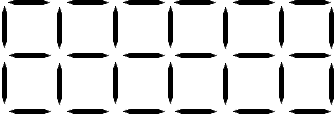# Toothpicks ActivityDirections:

1. Use toothpicks to make the pattern.
2. Select a strategy to find how many tooth picks are in the pattern without counting each toothpick?
3. Write an equation to illustrate a strategy to determine the number of tooth picks. You may change your strategy.
4. Exchange an equation with a partner. Prove to yourself the strategy you were given will determine the number of toothpicks.
5. Return the equation to the partner and explain strategies to each other.
6. Form groups of four and repeat steps 5 and 6.
7. Everyone puts their equation on the board.
8. Each person tries to understand how each equation on the board is a strategy to find a solution.
9. Take turns listening and explaining the equations on the board until everyone understands the strategy for each.
10. Organize the equations into categories.
11. Each person, pair, or group of four creates a new problem using what was learned today.
12. Each person or group shares a new problem with the others.
13. Which equation was the most unique? Why?
14. Each person or group shares what equation they think is most unique and why it's most unique with the others.

Hint: Look for patterns.
For example 6 C's (each C made with 3 toothpicks) and one toothpick at the end of the row make the top row of squares. Bottom row 6 L's plus one.

Discussion: Organize all the equations in some kind of order.

Mind boggler:

Is this a function or an equation or both?

Equation. x + 3 = 10; only one solution. An equation is only one way to express a relationship.

Function. For F(x) there is only one solution (unique answer). A function is a relationship between two variables such that every value of the independent variable, within the domain of the function, maps to one value for the dependent variable.

Functions can pass a verticle line test (a visual way to determine if a curve is a graph of a function or not by seeing if the line passes through multiple y points above the same point on x. If it does, then there are more than one solution. For example, a graph in the shape of the letter S would have three points above one or more of the same x point.

Both Equation and Function. x = 2y + 3

Not all functions can be expressed in any familiar way as an equation, but all functions can be used to make equations.

Enjoy!